There was a recent article on dev.to that inspired me to take a walk down memory lane and recall some fond memories from my early days of coding.

When I was in high school, one of the very first programming languages I was introduced to was Logo. It was interactive and visual: with basic movement commands, you could have your cursor (“turtle”) draw basic shapes and intricate patterns. It was a great way to introduce the compelling concept of an “algorithm”: a series of instructions for a computer to execute.

Fortunately, the Logo programming language is available today as a Python package. So let’s jump right in, and you can discover the possibilities with Logo as we go along.

## Installing the Turtle package

Logo is available as the `turtle` package for Python. It’s part of the Python Standard Library so there’s no need to install it separately.

The package relies on `tkinter` for the graphics which does need to be installed. If you run into `ModuleNotFoundError: No module named '_tkinter'`, you can install the library using:

``````# Ubuntu
sudo apt-get install python3-tk

# Mac OS X
brew install python-tk
``````

### Bob draws a square

With the `turtle` package installed, let’s draw some basic shapes.

To draw a square, imagine a turtle (let’s call him Bob) in the middle of your screen, holding a pen with his tail. Every time Bob moves, he draws a line behind him. How should Bob move to draw a square?

1. Move forward 100 steps.
2. Turn right 90 degrees.
3. Move forward 100 steps.
4. Turn right 90 degrees.
5. Move forward 100 steps.
6. Turn right 90 degrees.
7. Move forward 100 steps.

Now let’s put the above algorithm in Python. Create a file called `logo.py` and place the following code in it.

``````import turtle

if __name__ == '__main__':

turtle.title('Hi! I\'m Bob the turtle!')
turtle.setup(width=800, height=800)

bob = turtle.Turtle(shape='turtle')
bob.color('orange')

# Drawing a square
bob.forward(100)
bob.right(90)
bob.forward(100)
bob.right(90)
bob.forward(100)
bob.right(90)
bob.forward(100)

turtle.exitonclick()
``````

Save the above as `logo.py` and run `python3 logo.py`. Bob will draw a square on the screen.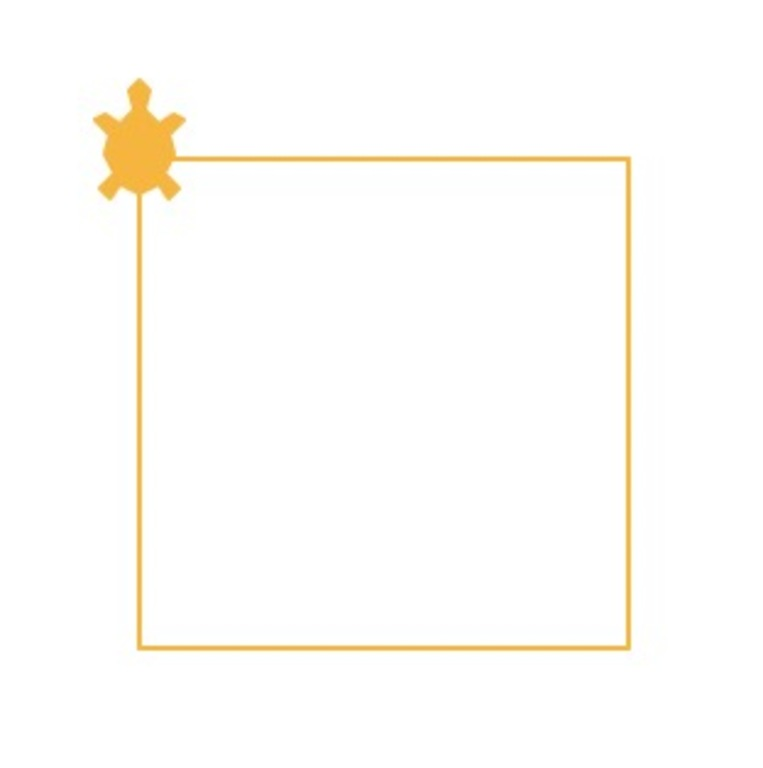Enlarge— Rediscovering Logo with Bob the turtle.

### Bob draws a hexagon

To draw a hexagon, Bob would need to move like this:

1. Move forward 150 steps.
2. Turn right 60 degrees.
3. Move forward 150 steps.
4. Turn right 60 degrees.
5. Move forward 150 steps.
6. Turn right 60 degrees.
7. Move forward 150 steps.
8. Turn right 60 degrees.
9. Move forward 150 steps.
10. Turn right 60 degrees.
11. Move forward 150 steps.

In Python, we can use a `for` loop to move Bob:

``````import turtle

if __name__ == '__main__':

turtle.title('Hi! I\'m Bob the turtle!')
turtle.setup(width=800, height=800)

bob = turtle.Turtle(shape='turtle')
bob.color('orange')

# Drawing a hexagon
for i in range(6):

bob.forward(150)
bob.right(60)

turtle.exitonclick()
``````

Run the above code with Python 3, and watch Bob draw a hexagon.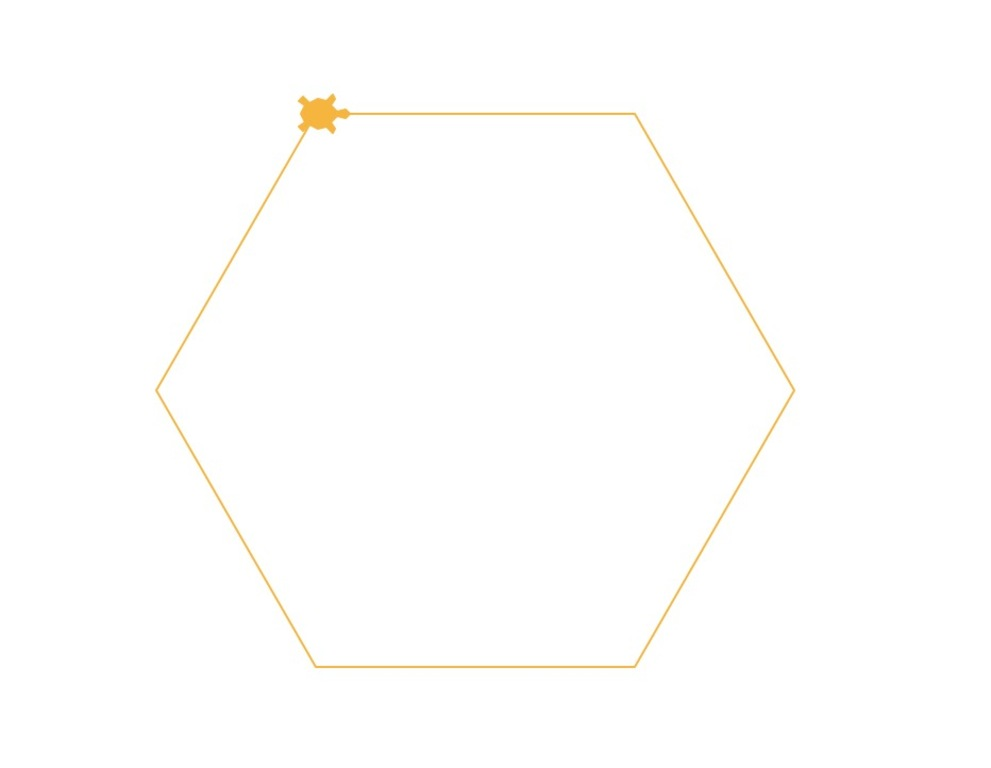Enlarge— Rediscovering Logo with Bob the turtle.

### Bob draws a square spiral

Now let’s draw a square spiral, but this time we’ll speed things up a bit. We can use the `speed` function and set `bob.speed(2000)` so that Bob moves faster.

``````import turtle

if __name__ == '__main__':

turtle.title('Hi! I\'m Bob the turtle!')
turtle.setup(width=800, height=800)

bob = turtle.Turtle(shape='turtle')
bob.color('orange')

# Drawing a square spiral
bob.speed(2000)
for i in range(500):

bob.forward(i)
bob.left(91)

turtle.exitonclick()
``````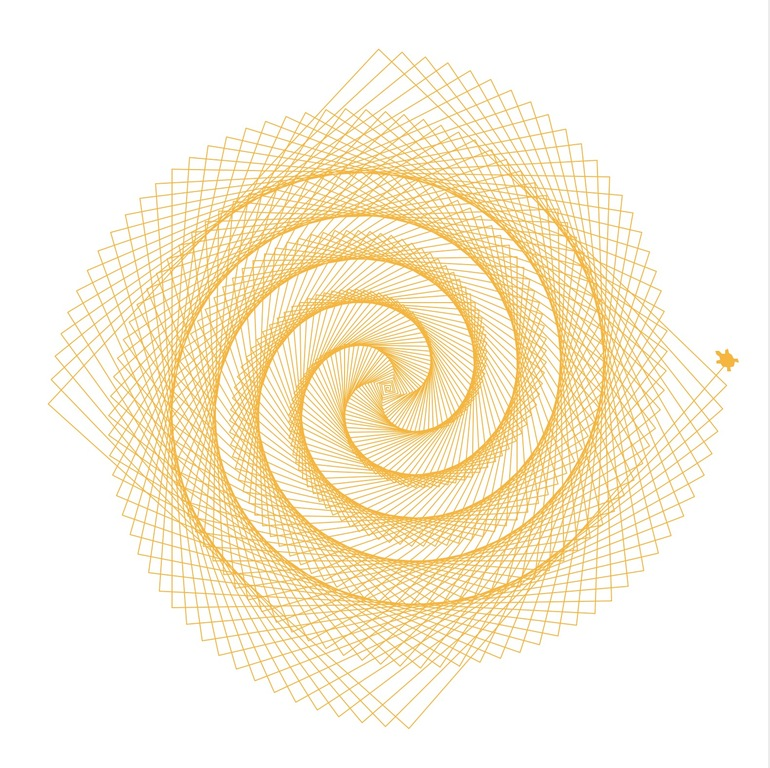Enlarge— Rediscovering Logo with Bob the turtle.

### Bob and Larry draw a weird snake thing

In the above examples, we initialised `Bob` as an object of the `Turtle` class. This time we’ll have another turtle, `Larry`, and they’ll be drawing together.

The `penup()` function makes the turtles lift their pens so they don’t draw anything as they move, and the `stamp()` function places a marker whenever it’s called.

``````import turtle

if __name__ == '__main__':

turtle.title('Hi! We\'re Bob and Larry!')
turtle.setup(width=800, height=800)

bob = turtle.Turtle(shape='turtle')
larry = turtle.Turtle(shape='turtle')
bob.color('orange')
larry.color('purple')

bob.penup()
larry.penup()
bob.goto(-180, 200)
larry.goto(-150, 200)
for i in range(30, -30, -1):

bob.stamp()
larry.stamp()
bob.right(i)
larry.right(i)
bob.forward(20)
larry.forward(20)

turtle.exitonclick()
``````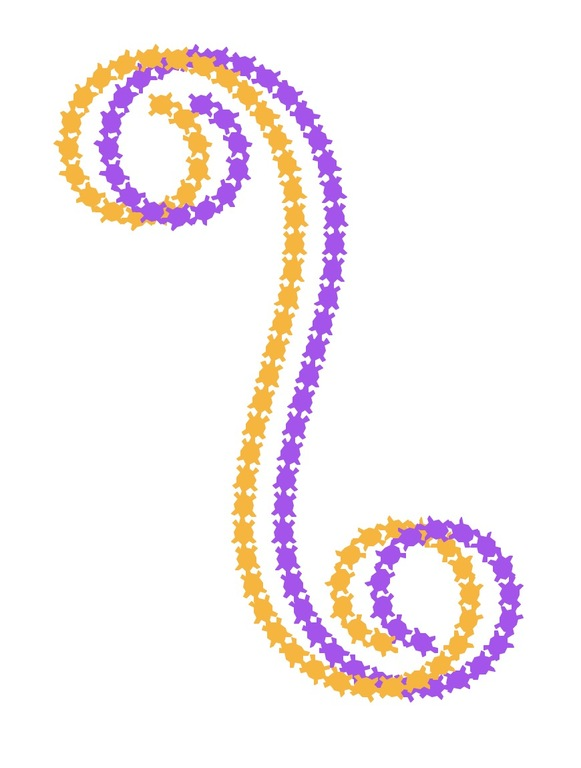Enlarge— Rediscovering Logo with Bob the turtle.

### Bob draws a sunburst

Bob can also draw simple lines and fill them in with colour. When we call the functions `begin_fill()` and `end_fill()`, Bob fills that shape with the colour set with `fillcolor()`.

``````import turtle

if __name__ == '__main__':

turtle.title('Hi! I\'m Bob the turtle!')
turtle.setup(width=800, height=800)

bob = turtle.Turtle(shape='turtle')
bob.color('orange')

# Drawing a filled star thingy
bob.speed(2000)
bob.fillcolor('yellow')
bob.pencolor('red')

for i in range(200):

bob.begin_fill()
bob.forward(300 - i)
bob.left(170)
bob.forward(300 - i)
bob.end_fill()

turtle.exitonclick()
``````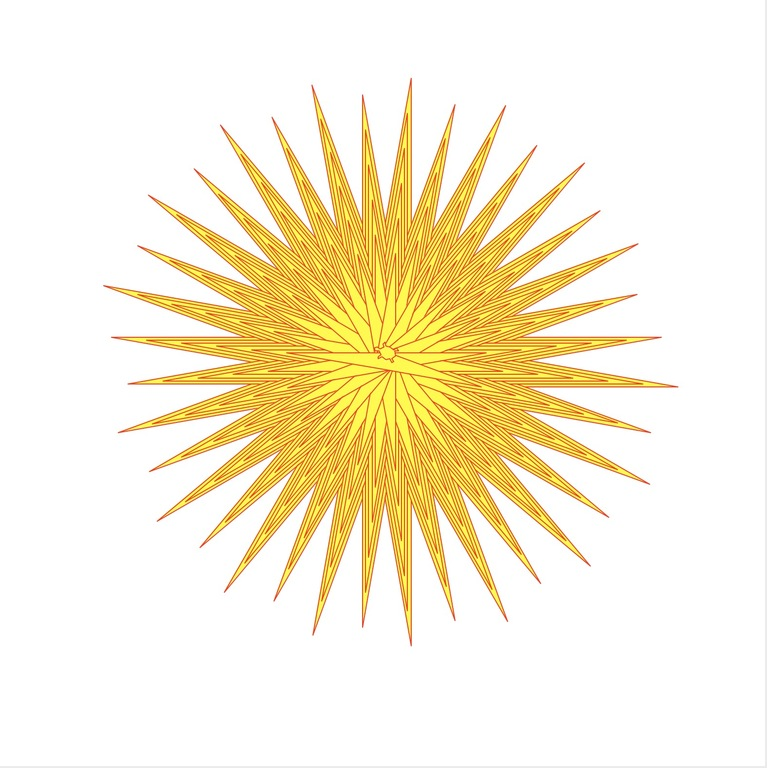Enlarge— Rediscovering Logo with Bob the turtle.

### Larry draws a Sierpinski triangle

Bob enjoys drawing simple geometrical shapes holding a pen with his tail as much as the next turtle, but what he enjoys most is drawing fractals.

One such shape is the Sierpinski triangle, which is an equilateral triangle recursively sub-divided into smaller equilateral triangles. It looks something like this: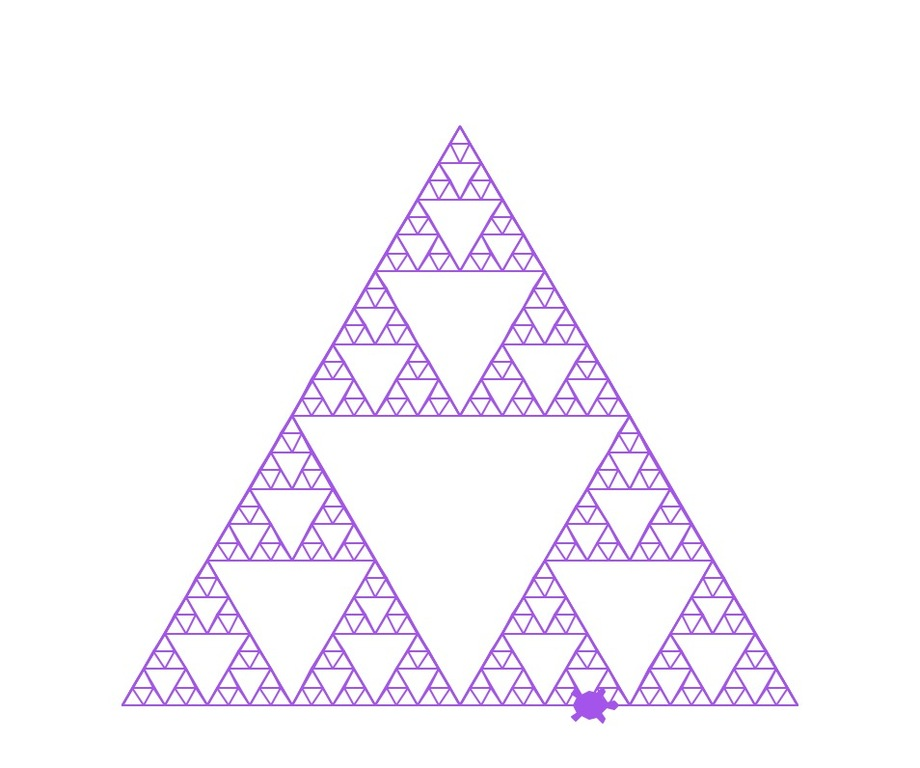Enlarge— Rediscovering Logo with Bob the turtle.

To draw the Sierpinski triangle above, Bob will have to work a bit harder:

``````import turtle

def get_mid_point(point_1: list, point_2: list):

return ((point_1 + point_2) / 2, (point_1 + point_2) / 2)

def triangle(turtle: turtle, points, depth):

turtle.penup()
turtle.goto(points, points)

turtle.pendown()
turtle.goto(points, points)
turtle.goto(points, points)
turtle.goto(points, points)

if depth > 0:

triangle(turtle, [points, get_mid_point(points, points), get_mid_point(points, points)], depth-1)
triangle(turtle, [points, get_mid_point(points, points), get_mid_point(points, points)], depth-1)
triangle(turtle, [points, get_mid_point(points, points), get_mid_point(points, points)], depth-1)

if __name__ == '__main__':

turtle.title('Hi! I\'m Bob the turtle!')
turtle.setup(width=800, height=800)

larry = turtle.Turtle(shape='turtle')
larry.color('purple')

points = [[-175, -125], [0, 175], [175, -125]]  # size of triangle

triangle(larry, points, 5)

turtle.exitonclick()
``````

The Logo programming language is a great way to teach basic programming concepts, such as how a computer can execute a set of commands. Also, since the library is now available in Python, it can be used to visualise more complex ideas and concepts.

I hope Bob and Larry have been enjoyable and instructive. Have fun, and happy coding :)